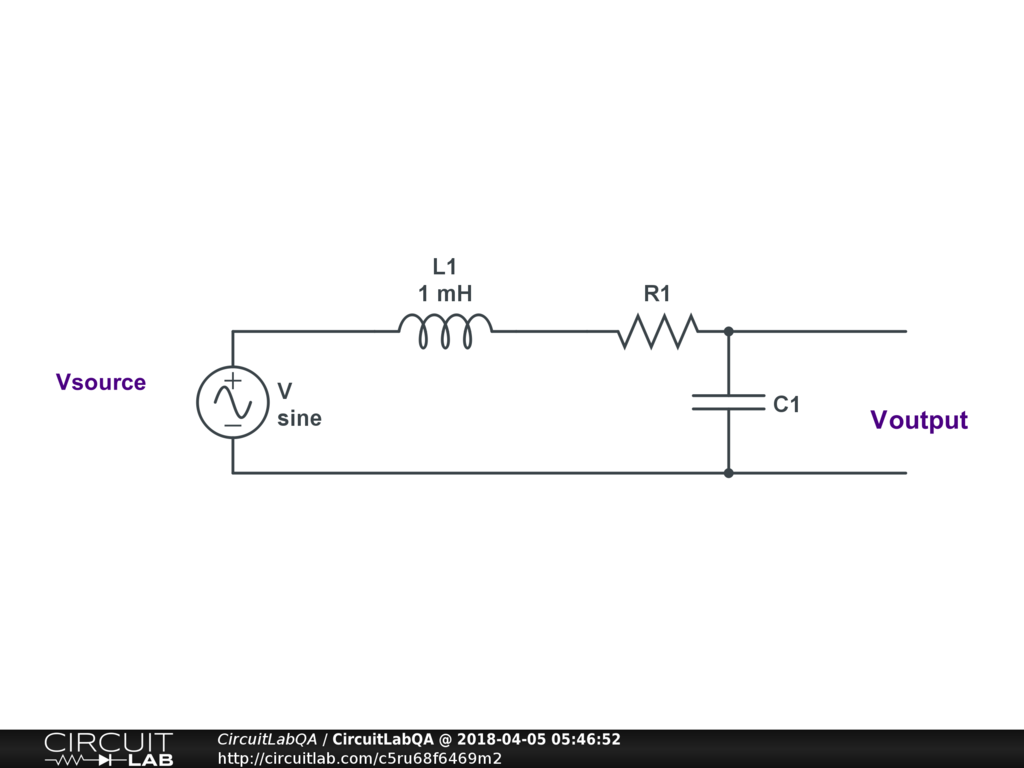## Passive Second Order Low-pass Circuit.Hi everyone. I am working on an assignment about passive second order low-pass filters. The circuit is attached. We are given the placements of the passive components (Inductor(L1), Resistor(R1) and Capacitor(C1)) without their values. The only given specification is that the cut-off frequency is 78 kHz. My questions are: 1.How to make the predicted low-pass filter Transfer function for this specific circuit? 2.Secondly how do I calculate or choose the component values for the filter? ... ( A suggestion given to me was to choose a Inductor value and a Capacitor value that is at my disposal ant to calculate the required Resistor value to build the circuit after calculations ). The components must work out so that: 2.1) the phase angle between the output voltage and input voltage is -90 degrees at the cut-off frequency (78 kHz). AND 2.2) that the ratio of the amplitudes of the Output Voltage to the amplitude of the Input Voltage is 0.7079 at the cut-off frequency. PS: I have a 1mH Inductor at my disposal,( thats why it is the only value I entered on the circuit for now :) ) and the Capacitors at my disposal is 1uF, 10uF, 22uF, 100uF or 470uF ). I can't wait to here from you! by iammichelle April 05, 2018 The circuit transfer response should be Xc/(Xc+Xr+Xl). Simply substitute for these and you will see how to calculate the circuit values. by mikerogerswsm April 05, 2018

You must log in or create an account (free!) to answer a question.

### Ask a Question

Anyone can ask a question.

Did you already search (see above) to see if a similar question has already been answered? If you can't find the answer, you may ask a question.

CircuitLab's Q&A site is a FREE questions and answers forum for electronics and electrical engineering students, hobbyists, and professionals.

We encourage you to use our built-in schematic & simulation software to add more detail to your questions and answers.

Acceptable Questions:

• Concept or theory questions
• Practical engineering questions
• “Homework” questions
• Software/hardware intersection
• Best practices
• Design choices & component selection
• Troubleshooting

Unacceptable Questions:

• Non-English language content
• Non-question discussion
• Non-electronics questions
• Vendor-specific topics
• Pure software questions
• CircuitLab software support

Please respect that there are both seasoned experts and total newbies here: please be nice, be constructive, and be specific!